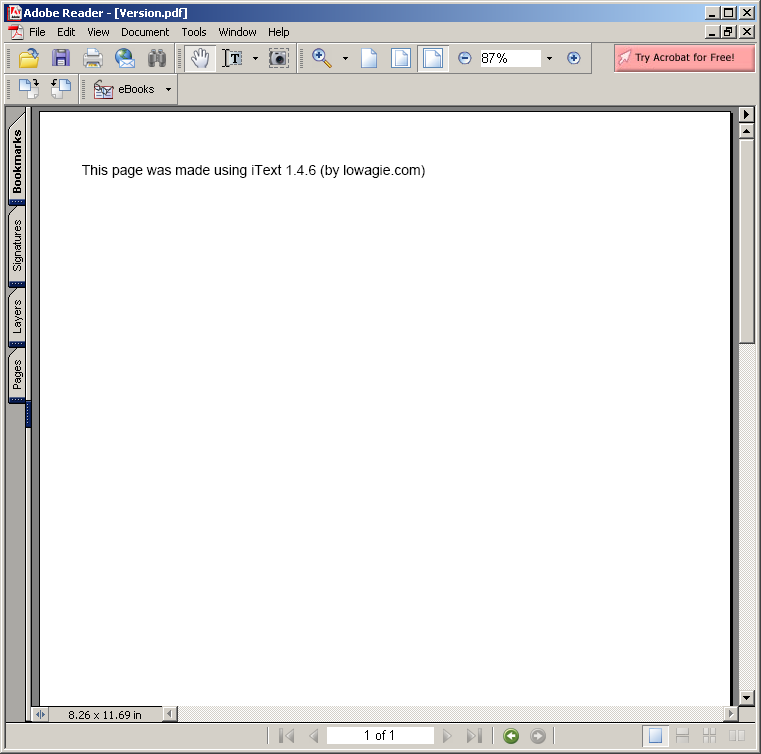# Compute the area of a triangle using Heron's Formula : Algorithms « Collections Data Structure « Java

Compute the area of a triangle using Heron's Formula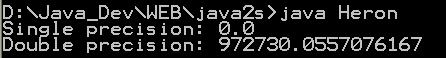```

/** Compute the area of a triangle using Heron's Formula.
* Code and values from Prof W. Kahan and Joseph D. Darcy.
* See http://www.cs.berkeley.edu/~wkahan/JAVAhurt.pdf.
* Derived from listing in Rick Grehan's Java Pro article (October 1999).
* Simplified and reformatted by Ian Darwin.
*/
public class Heron {
public static void main(String[] args) {
// Sides for triangle in float
float af, bf, cf;
float sf, areaf;

// Ditto in double
double ad, bd, cd;

// Area of triangle in float
af = 12345679.0f;
bf = 12345678.0f;
cf = 1.01233995f;

sf = (af+bf+cf)/2.0f;
areaf = (float)Math.sqrt(sf * (sf - af) * (sf - bf) * (sf - cf));
System.out.println("Single precision: " + areaf);

// Area of triangle in double
bd = 12345678.0;
cd = 1.01233995;

aread =        Math.sqrt(sd * (sd - ad) * (sd - bd) * (sd - cd));
System.out.println("Double precision: " + aread);
}
}

```

### Related examples in the same category

 1 Anagrams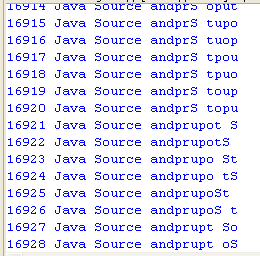2 Hanoi puzzle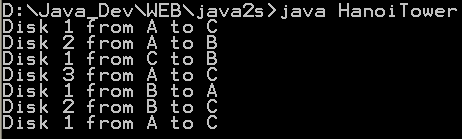3 Fibonacci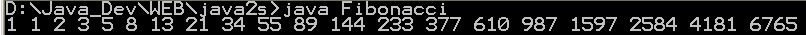4 Sieve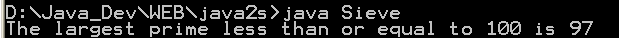5 Find connections using a depth-first search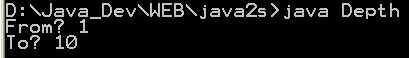6 Find connections using hill climbing. 7 Find optimal solution using least-cost 8 Find the lost keys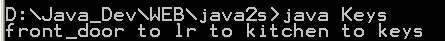9 Compute prime numbers 10 Print a table of fahrenheit and celsius temperatures 1 11 Print a table of fahrenheit and celsius temperatures 2 12 Print a table of Fahrenheit and Celsius temperatures 3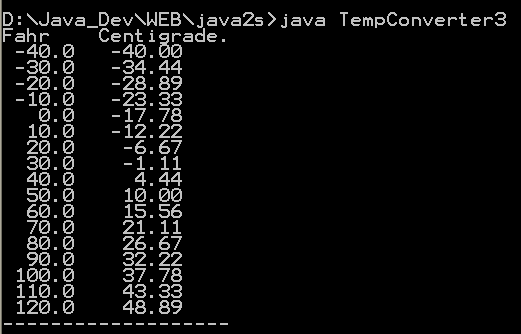13 Soundex - the Soundex Algorithm, as described by Knuth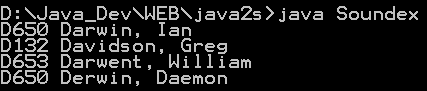14 A programmable Finite State Machine implementation. 15 An extendable Graph datastructure. 16 Utilities for flop (floating-point operation) counting. 17 LU Decomposition 18 Reverse Polish Notation 19 Permutator test 20 implements the LZF lossless data compression algorithm 21 Linear Interpolation 22 Utility class for generating the k-subsets of the numbers 0 to n 23 Version